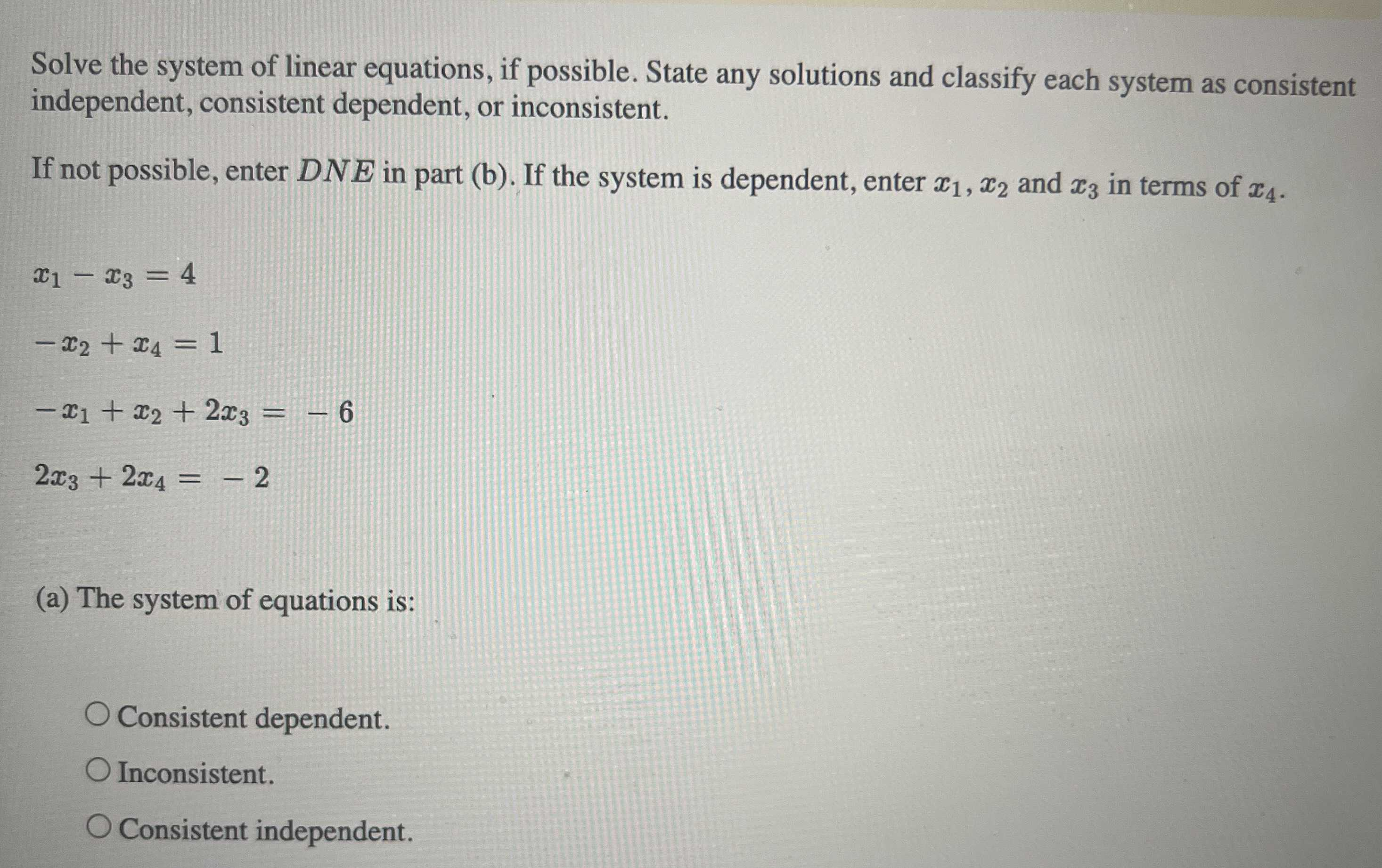### Still have math questions?

Algebra
QuestionSolve the system of linear equations, if possible. State any solutions and classify each system as consistent independent, consistent dependent, or inconsistent. If not possible, enter $$D N E$$ in part (b). If the system is dependent, enter $$x _ { 1 } , x _ { 2 }$$ and $$x _ { 3 }$$ in terms of $$x _ { 4 }$$ .

$$x _ { 1 } - x _ { 3 } = 4$$

$$- x _ { 2 } + x _ { 4 } = 1$$

$$- x _ { 1 } + x _ { 2 } + 2 x _ { 3 } = - 6$$

$$2 x _ { 3 } + 2 x _ { 4 } = - 2$$

(a) The system of equations is: